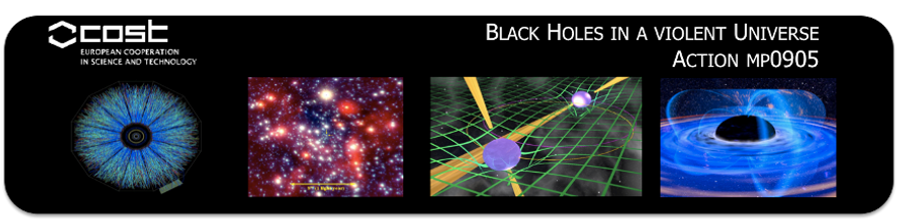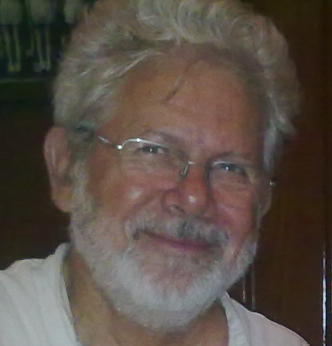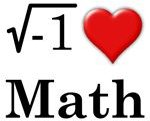# Report on "From classical to quantum GR: applications to black holes"I attended the winter school “From classical to quantum GR: applications to black holes” at the University of Sussex. The school was for three days over the 16th, 17th and 18th of January 2013.

The school was organised within the COST action black holes in a violent universe MP0905. You can find out more about the action here. The local organiser was Dr Xavier Calmet, who did a good job.

The school covered a mixture of theoretical physics, phenomenology and observational astronomy. The program consisted of reviews of general relativity, quantum gravity, astroparticle physics and black hole physics. The main emphasis of the lectures was on how to probe new gravitational effects using colliders and astrophysical observations.Image courtesy of NASA (concept drawing)

Due to the wide range of speakers, the level and tone of the lectures was kept informal. Indeed, a large proportion of the audience were PhD and masters students. from my point of view the level of the lectures was right, black hole physics is not my area of expertise.

Overall I enjoyed the school and the friendly atmosphere at Sussex.

The lectures
The lectures were for 3 hours, broken up into two parts. That sounds quite monstrous, but the pedagogical nature of the talks tempered this. The quality of the lectures was very high.

The speakers were

• David Champion (Max-Planck-Institut fuer Radioastronomie)
• Panagiota Kanti (University of Ioannina)
• Claus Kiefer (Universitaet zu Koeln)
• Iossif Papadakis (University of Crete)
• Eram Rizvi (Queen Mary, University of London)
• Elizabeth Winstanley (University of Sheffield)

A timetable of the lectures can be found here.

I believe slides will be available at some point. I will link to them in due course.Simulated view of a black hole in front of the Large Magellanic Cloud.

Snow
Heavy snow fell on the Friday, so the winter school really did feel like a winter school!

Funding

The main source of funding was the COST action. You can find out more about COST here.

Further financial support was given by the Institute of Physics and in particular from its High Energy Particle Physics group and Mathematical and Theoretical Physics Group.

COST
COST, “European Cooperation in Science and Technology”, is an intergovernmental framework for European Cooperation in Science and Technology, allowing the coordination of nationally-funded research on a European level.

Find out more here.

Theoretical Particle Physics group at Sussex

IOP High Energy Particle Physics Group

IOP Mathematical and Theoretical Physics Group

# IOP Newton Lecture, 'From Mars to the Multiverse', 28 February

From Mars to the Multiverse

Prof Martin Rees, Lord Rees of Ludlow

Institute of Astronomy

Date: Thursday 28 February 2013

Venue: Institute of Physics, 76 Portland Place, London, W1B 1NT

Time: 17.30 (registration from 17.00)Prof Martin Rees

‘Astronomers have made astonishing progress in probing our cosmic environment. We can trace cosmic history from some mysterious “beginning” nearly 14 billion years ago, and understand in outline the emergence of atoms, galaxies, stars and planets.

But the key parameters of our expanding universe — the expansion rate, the geometry and the content — were established far earlier still, when the physics is still conjectural but can be pinned down by future observations. These advances pose new questions: What does the long-range future hold? Should we be surprised that the physical laws permitted the emergence of complexity? And is physical reality even more extensive than the domain that our telescopes can probe? This illustrated lecture will attempt to address such issues.’

Note
The original message is from Claire Copeland, Science Support Officer at the Institute of Physics.

# No recovery in the number of university applications

The worrying trend of falling university applications continues, according to the most recent statistics published by UCAS. We urge the Government to step in with a national campaign to promote the value of university for potential students currently considering their options, whether they are about to leave school or considering a university course later in life.

Pam Tatlow, Chief Executive of the university think tank, million+

University applications for fulltime courses for the academic year 2013/14 submitted by 17 December were published by UCAS on 3 January.

Highlights

1. The published figures highlight that there is an overall fall in applications compared to 2012/13 of -5.6%, and a fall of -6.5% in England.
2. Excluding early applications which were submitted by 15 October for Oxbridge, medicine, dentistry and veterinary courses, applications are down by 7.2%, and 7.6% in England.
3. Admissions in 2012/13 were already down on previous years, with 54,000 fewer people starting university courses compared to 2011/12.

You can find out more here.

Million +

The latest High Fliers study suggests that top employers plan to hire 18,306 graduates in 2013, some 2.7% more than last year.

In addition employers will provide paid work experience places for 11,387 students and new graduates.

Good news for recent graduates following a few years of general bad news.

# The derived product on a Q-manifold

This went “under my radar” for a while, I am not sure why, but anyway…

Q-manifolds
Recall that a Q-manifold is a supermanifold $$M$$ equipped with a homological vector field, that is an odd vector field $$Q$$ such that $$[Q,Q]=2 Q^{2}=0$$.

The algebra of functions $$C^{\infty}(M)$$ is thus a supercommutative differential algebra. The product of two functions is again a function and importantly this is supercommutative:

$$f g = (-1)^{\widetilde{f} \widetilde{g} } g f$$,

where I denote the Grassmann parity of a homogeneous function by the “tilde”. Just as on a classical manifold, the product of two functions is associative:

$$f(gh) = (fg)h$$,

for all functions f,g,h. Also important is that the product itself does not carry any Grassmann parity, thus

$$\widetilde{fg} = \widetilde{f} + \widetilde{g}$$.

The prime example here is to take $$M = \Pi TN$$ where $$N$$ is a classical manifold. The functions $$C^{\infty}(\Pi TM)$$ are identified with (pseudo)differential forms and the homological vector field is just the de Rham differential.

The derived product

Definition Let $$(M,Q)$$ be a Q-manifold. Then the derived product on $$C^{\infty}(M)$$ is defined as

$$f \ast g = (-1)^{\widetilde{f}+1} Q(f)g$$.

1. The derived product is Grassmann odd, $$\widetilde{f\ast g} = \widetilde{f} + \widetilde{g}+1$$
2. The derived product is clearly not supercommutative

Remark One can consider a derived product on any differential algebra, one does not need the supercommutivity, but as I am interested in supergeometry the definition here suits well.

Right away the derived product seems a strange beast, it carries Grassmann parity itself. Being noncommutative is also interesting, but we are used to noncommutative forms of multiplication.

Theorem The derived product is associative.

Proof Explicitly
$$f \ast (g \ast h) = (-1)^{\widetilde{f} + \widetilde{g}} Q(f)Q(g)h$$.

Now consider

$$(f \ast g)\ast h = (-1)^{\widetilde{f} + \widetilde{g}}Q ((-1)^{\widetilde{f}+1}Q(f)g )h$$.

Using the fact that $$Q$$ is homological, that is odd and squares to zero we get

$$(f \ast g)\ast h =(-1)^{\widetilde{f} + \widetilde{g}} Q(f)Q(g) h$$.

QED

Theorem $$f \ast g = (-1)^{(\widetilde{f}+1)(\widetilde{g}+1)} g \ast f + (-1)^{\widetilde{f}+1}Q(fg)$$.

Proof Left as an exercise for the readers.

The above theorem shows very explicitly that the derived product is not supercommutative. It is however antisymmetric if and only if $$Q(fg)=0$$.

Where has this come from?
As far as I am aware, the notion of a derived product can be found in the work of Loday;

J.L. Loday. Dialgebras. In Dialgebras and related operads, 7-66, Lecture Notes in Math., 1763, Springer, Berlin 2001.Jean-Louis Loday,
(12 January 1946 – 6 June 2012)

Concluding remarks
We have a kind of noncommutative deformation of the algebra of functions on a Q-manifold via the derived product. This “new product” carries Grassman parity and is associative, it is closer to the standard notion of a product than say a Lie algebra.

I am sure there is lots more to say about derived products, but this is something I am only just beginning to explore. Watch this space…

# The idea of a proof

In an earlier post I talked about the notions of a lemma, a theorem, a proposition and a corollary. There is in truth, not much difference. So, now I want to say a few informal words about the notion of a proof.

Proof
A proof is a demonstration that if some statements are true, or assumed to be true (i.e. axioms), then some other mathematical statement or statements are necessarily true.

A proof is based on deductive reasoning; if “something” then “something”. All proofs employ logic, though most proofs in mathematics will employ informal logic, rather than formal logic which is the subject of proof theory, a subject I know little about.

The rigor and style of a proof will vary mathematician to mathematician as well as subject to subject. The main thing is if you produce a proof of some statement in some area of mathematics then it should be of a style and at the level of rigor accepted by the mathematicians working in that area.

The key thing about a proof is that it should cover all cases as specified by the statement. “Proofs” that establish the statement for only a fraction of the possible cases will not establish the statement for all cases.

Once a statement is proved it is rather “cast in stone”. It is an unshakable fact that is absolutely true now, as well as always having been true and will always be true.

That all said, mathematicians are human and mistakes can creep in as well as loopholes due to the level of rigor. Worse than that we have Gödel’s incompleteness theorems, but lets forget all about that and another possible complications.

Inductive reasoning
Proofs of statements follow from previously established statements or statements taken to be true.

Discovering a new statement does not always follow in a deductive fashion from earlier statements, though the full proof will. Often the reasoning is more inductive following the creation of specific examples and weakening restrictions on earlier statements.

The discovery of new mathematics can be inductive, but the presentation of new mathematics is usually deductive.

Examples
Finding examples to show that a statement is true, is in my opinion quite important. Also counter examples can be very illustrative. One can certainly build a picture of what is going on that way and build confidence in ones ideas, but only an exhaustive presentation of all the possible cases would constitute a proof. In practice this can simply be impossible; for example one could not prove a theorem on prime numbers that way as there is an infinite number of prime numbers!

An example where an exhaustive exploration of all possible cases is possible is the four colour theorem which has 1,936 cases. The theorem was proved in 1976 by Kenneth Appel and Wolfgang Haken using a computer. There proof is the first proof constructed using a computer that has been generally accepted.

Constructive and nonconstructive proofs
A proof of some statement may just constitute the establishment that a required mathematical object exists. The proof may not give you any indication about how to construct such an object: these proofs are usually to do with “pure existence theorems”.

This is the difference between constructive and nonconstructive proofs. The first gives you the required object explicitly, while the latter only establishes that such an object exists.

One can then argue how “useful” a nonconstructive proof is if you cannot actually find the object required. That said, one may only really need to know that it exists to establish further mathematics.

A proof should be illustrative
Hopefully you have a watertight proof of some statement. That is the main thing, however a proof should also make it clear why the statement is true. Not only will this make your statement clearer, but also it might allow further generalisations of your statement very directly.

Final remarks
There is a lot more to proofs than I have said here, there is a whole branch of logic devoted to the notion of proof. For most working mathematicians proof theory, as it is known, would be “overkill”. Most proofs are, in accordance with proof theory, informal proofs which employ some logic and natural language, but not the full machinery of logic.Public domain image by Peter Valberg It is probably worth comparing mathematics with the empirical sciences with the notion of a proof in mind, but that is a post for another time.

# Heidelberg Laureate ForumThe first Heidelberg Laureate Forum is going to place on September 22-27, 2013. The forum aims to bring together the best students and early stage researchers in mathematics & computer science with winners of the most prestigious awards in these disciplines – Abel, Fields, and Turing Laureates.

Applications to attend must be submitted by the 15th February 2013. Please see the website below for details.

Heidelberg Laureate Forum

# Lemma, Theorem, Proposition or Corollary?

A student recently ask me about to explain what mathematicians mean by a corollary, so I thought I would quickly explain here.Image by Free-StockPhotos.com

The four labels given by mathematicians to statements that can be shown to be true are Lemma, Theorem, Proposition and Corollary. They all basically mean the same thing: some mathematical statement that is true, given some starting axioms or previous true statements. Showing that these statements are true constitutes a proof. I will say something about proofs another time.

Everything I say here will be rather informal.

Lemma

A lemma is some statement that can be shown to be true, starting from some previously accepted statements, that is used to show that some other statement or statements are true.

In essence, a lemma is a “stepping stone” to some other statement or statements that are regarded more important in the current context.

However, lemmas can turn out to be very important statements in many cases. They often develop some line of attack that can be applied to a wider context than they were originally intended. Some of the most famous results in mathematics are lemmas.

Theorem

A theorem is some statement that can be shown to be true, starting from some previously accepted statements.

This is almost the same as a lemma, but a theorem is deemed to be of primary importance within the context it is established. Though note that one theorem can be used to prove another theorem, so the distinction between lemma and theorem is loose.

You can find a list of theorems here.

Proposition

A proposition is some statement that can be shown to be true, starting from some previously accepted statements.

Hang on a moment, is this not just the same as a theorem?

In short, yes a theorem and a proposition are the same thing. Though, and this is rather subjective, a theorem is deemed to be a more important result than a proposition.

So the distinction between a lemma, a theorem and a proposition is rather loose.

Corollary

A corollary is some statement that is true, that follows directly from some already established true statement or statements.

Typically, a corollary will be some statement that is easily derived from a theorem or a proposition. Often corollaries are “specialisations” of a theorem or a proposition. They key thing is they follow naturally from some established statement.

But again, this is not really any different to a theorem or proposition, or indeed a lemma if the corollary is used to establish some other statements.

In conclusion

So we see that the distinction between a lemma, a theorem, a proposition and a corollary is not very strict and depends largely on the context as well as the whims of the mathematicians writing papers.

One man’s lemma is another man’s theorem.

# The University of Buckingham suspends accreditation for courses at the Victoria University in Uganda

The University of Buckingham,  which is the only private research and teaching university of the United Kingdom, is to stop accreditation of courses run at the Victoria University in  Kampala, Uganda.

This is in direct response to proposed legislation against homosexuality in Uganda. Homosexuality is already against the law in Uganda, but the new bill would increase the punishment, including the possibility of a life sentence.

Under both UK and Ugandan law discrimination on a variety of grounds is prohibited; however there are fundamental differences between the two nations’ respective laws regarding equality and diversity, which cannot be reconciled. After seeking legal guidance from both UK and Ugandan lawyers, Victoria University and University of Buckingham have concluded that as the laws of Uganda and UK presently stand, Victoria University cannot comply with both sets of laws.

Victoria University Press Release 8 Jan 2013

BBC News

# Not quite terminator the musical, but…

Not quite terminator the musical, but it is a start!